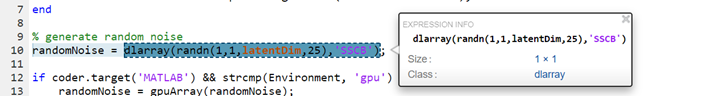dlarray Limitations for Code Generation

Recommended Usage

For code generation, use the dlarray (Deep Learning Toolbox) function to create deep learning arrays. For example, suppose you have a pretrained dlnetwork (Deep Learning Toolbox) network object in the mynet.mat MAT-file. To predict the responses for this network, create an entry-point function in MATLAB® as shown in this code.

function a = foo(in)
dlIn = dlarray(in, 'SSC');

persistent dlnet;
if isempty(dlnet)
end

dlA = predict(dlnet, dlIn);

a = extractdata(dlA);

end

Limitations

For deep learning arrays, code generation has the following limitations:

• The data format argument of the dlarray object must be a compile-time constant. For example,

function out = foo()

dlA = dlarray(ones(5,4),'SSC'); %fmt 'SSC' is constant
.
.
.
end

• The data input to the dlarray object must be fixed-size. For example, the dlarray dlA is not supported as A is variable-sized.

function dlA = foo()

A = ones(5,4);
coder.varsize('A') %'A' is variable sized.

dlA = dlarray(A, 'SSC'); % Error: not supported.

end

• Code generation does not support creating a dlarray type object by using the coder.typeof function with upper bound size and variable dimensions specified. For example,

function dlA = foo()

A = dlarray(ones(5,4),'SC');
A_type = coder.typeof(A,[5 10],[1 0]); % Error: not supported.

end

Code generation supports use of coder.typeof without the size arguments. For example,

A = dlarray(ones(5,4),'SC');
A_type = coder.typeof(A);

• The code generation report does not display the size of the dlarray object. The size is always displayed as 1x1.• In MATLAB, dlarray enforces the order of labels 'SCBTU'. This enforcement eliminates ambiguous semantics in operations, which implicitly match labels between inputs. This behavior is mimicked during MEX code generation. However, for standalone code generation such as static, dynamic libraries, or executables, the data format follows the specification of the fmt argument of the dlarray object. As a result, if the input or output of an entry-point function is a dlarray object and its order of labels is not 'SCBTU', then the data layout will be different between the MATLAB environment and standalone code.

For example, consider a function foo with a dlarray object as an output.

function dlA = foo()
rng default
dlA = dlarray(rand(5,4), 'BC');

end

In MATLAB, dlA is 4(C)-by-5(B).

dlA =

4(C) × 5(B) dlarray

0.8147    0.9058    0.1270    0.9134    0.6324
0.0975    0.2785    0.5469    0.9575    0.9649
0.1576    0.9706    0.9572    0.4854    0.8003
0.1419    0.4218    0.9157    0.7922    0.9595

For standalone code generation, dlA is 5(B)-by-4(C).

• For code generation, the dlarray input to the predict method of the dlnetwork object must be single data type.